# JAVA之旅（二十三）——System，RunTime，Date，Calendar，Math的数学运算JAVA之旅（二十三）——System，RunTime，Date，Calendar，Math的数学运算http://www.bieryun.com/2813.html## 一.System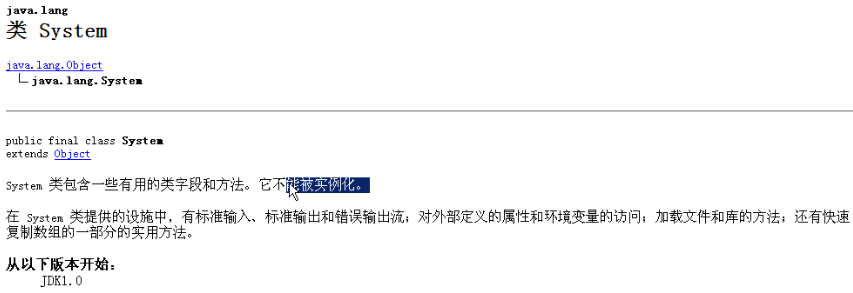``````package com.lgl.hellojava;

import java.util.Properties;

public class HelloJJAVA {
public static void main(String[] args) {
/**
* 描述系统的一些信息 获取系统的一些信息 ：
* Properties = getProperties
* out：标准输出，默认是控制台
* in:标准输入，默认控制台
*/

Properties properties = System.getProperties();
/**
* 因为Properties是HashTab的子类，也就是map集合的一个子类对象
* 那么可以用map的方法取出集合中的元素,该集合存储中都是字符串，
* 没有泛型定义
*/

for (Object obj : properties.keySet()) {
String value = (String) properties.get(obj);
System.out.println(obj + ":" + value);
}

}
}
``````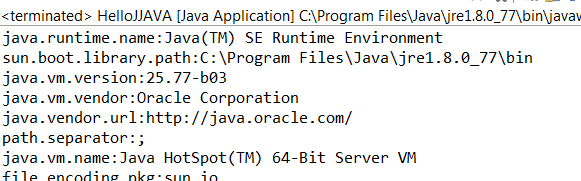``````        /**
* 如何在系统中自定义一些特有信息
*/
System.setProperty("mykey", "myvalue");
``````

``````package com.lgl.hellojava;

public class HelloJJAVA {
public static void main(String[] args) {

/**
* 获取指定属性信息
*/
String property = System.getProperty("os.name");
System.out.println(property);

}
}
``````

OK,就能获取到系统名字了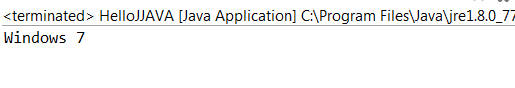## 二.RunTime

``````package com.lgl.hellojava;

import java.io.IOException;

public class HelloJJAVA {
public static void main(String[] args) {

Runtime r = Runtime.getRuntime();
try {
r.exec("C:\\Program Files\\Git\\git-bash");
} catch (IOException e) {
// TODO Auto-generated catch block
e.printStackTrace();
}

}
}
``````

## 三.Date

``````package com.lgl.hellojava;

import java.util.Date;

public class HelloJJAVA {
public static void main(String[] args) {
Date date = new Date();
System.out.println(date);
}
}
``````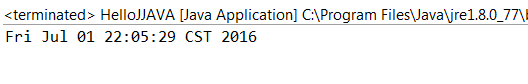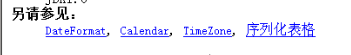``````package com.lgl.hellojava;

import java.text.SimpleDateFormat;
import java.util.Date;

public class HelloJJAVA {
public static void main(String[] args) {
Date date = new Date();
//将模式封装
SimpleDateFormat format = new SimpleDateFormat("yyyy年MM月dd日 hh:mm:ss");
//格式化Date对象
String time = format.format(date);
System.out.println(time);
}
}
``````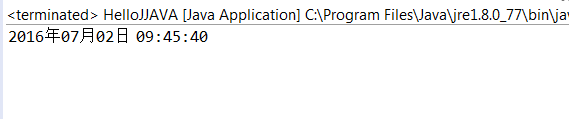## 四.Calendar

``````package com.lgl.hellojava;

import java.util.Calendar;

public class HelloJJAVA {
public static void main(String[] args) {

Calendar calendar = Calendar.getInstance();
String[] mons = { "一月", "二月", "三月", "四月", "五月", "六月", "七月", "八月", "九月",
"十月", "十一月", "十二月" };
int index = calendar.get(Calendar.MONTH);

//查询当前日期
sop(calendar.get(Calendar.YEAR) + "年");
sop((calendar.get(Calendar.MONTH) + 1) + "月");
sop(mons[index]);
sop(calendar.get(Calendar.DAY_OF_MONTH) + "日");
sop("星期：" + calendar.get(Calendar.DAY_OF_WEEK));

}

public static void sop(Object obj) {
System.out.println(obj);
}
}
``````

OK，这样就可以获取到了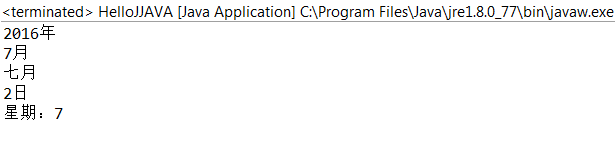## 五.Math

### 1.ceil

``````//返回大于指定数据的最小整数
double ceil = Math.ceil(12.34);``````

### 2.floor

``````//返回小于指定数据的最小整数
double ceil1 = Math.floor(12.34);
sop(ceil1);``````

### 3.round

``````//四舍五入
long ceil2 = Math.round(12.34);
sop(ceil2);``````

### 4.pow

``````// 2的3次方
double ceil3 = Math.pow(2, 3);
sop(ceil3);``````

### 5.random

``````/ 随机数
int ceil4 = (int) (Math.random()*10);
sop(ceil4);``````

``````Random r = new Random();
sop(r.nextInt(10));``````• 回顶